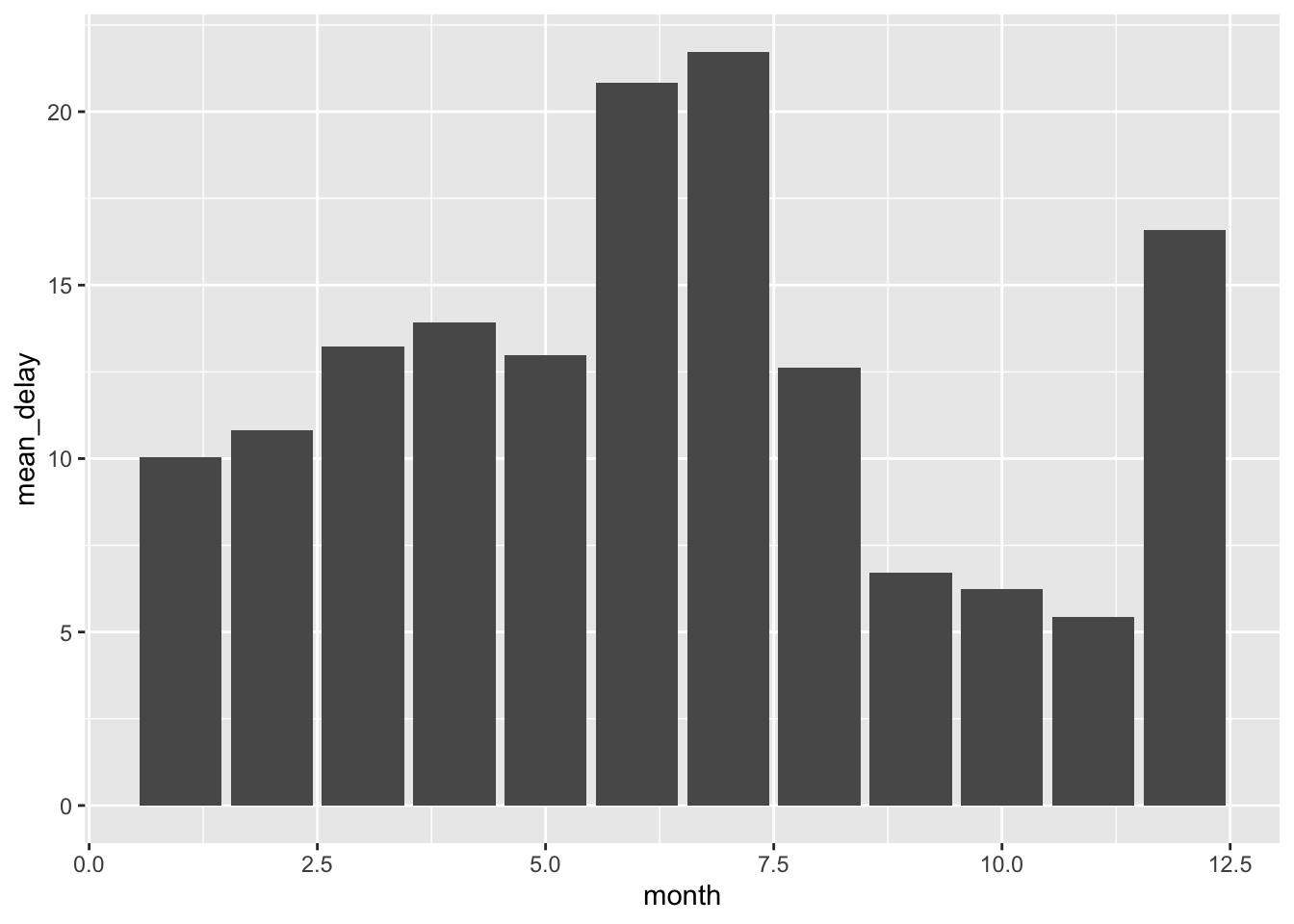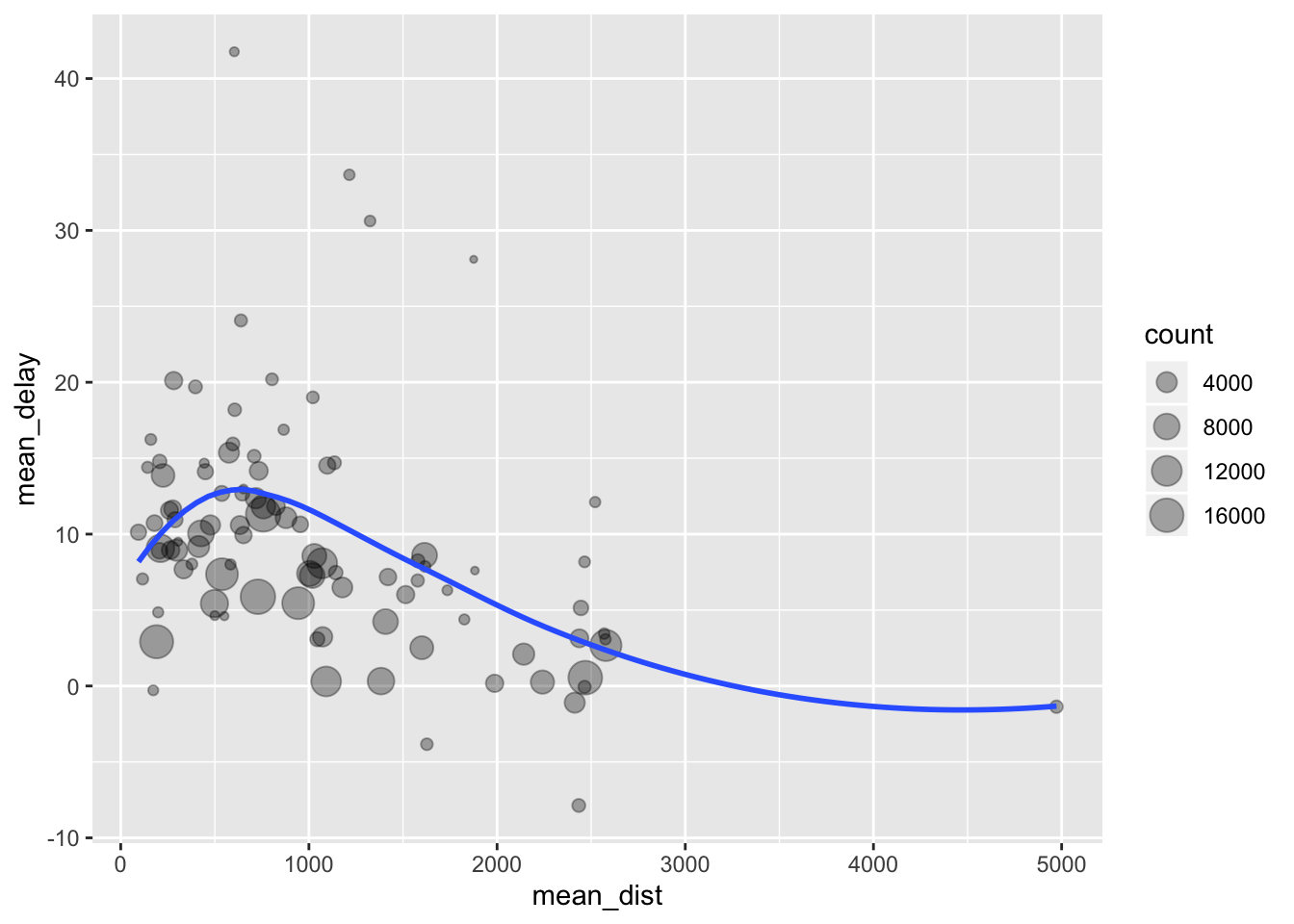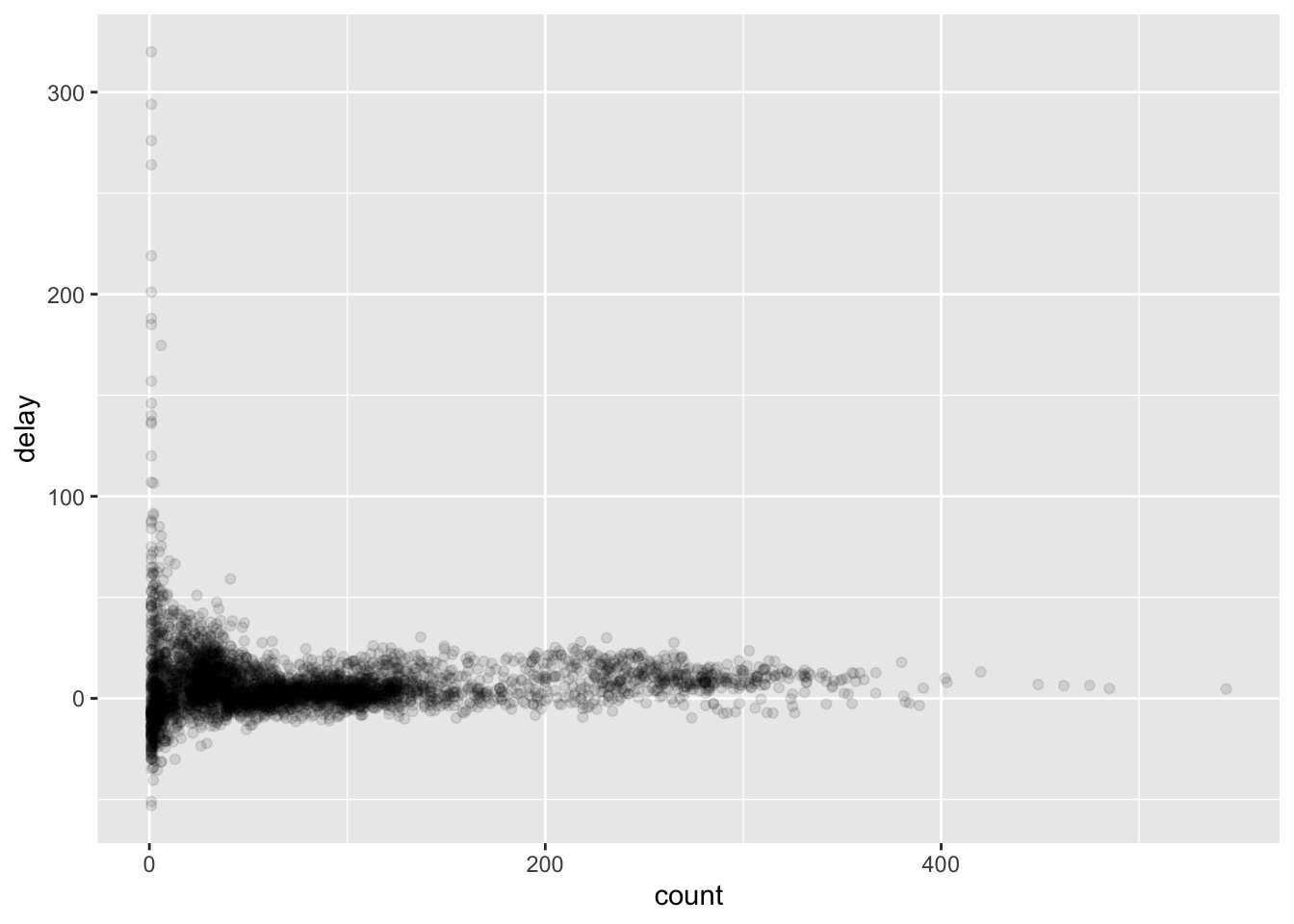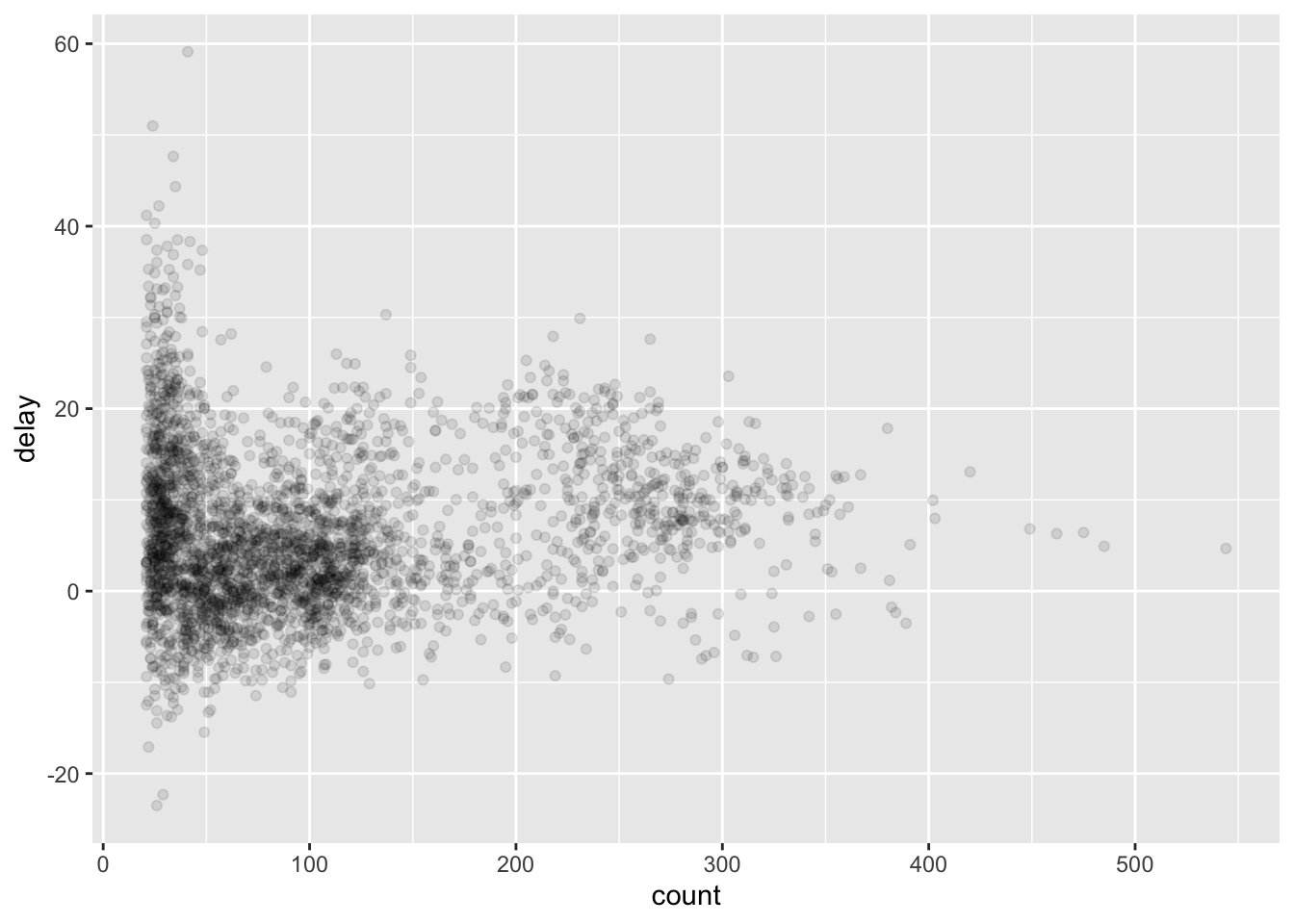# R Tutorial (4)

In this post, we will continue our journey down the R road to take a deeper dive into data frames. R is great for data analysis and wranging when it comes to dealing with tabular data, especially thanks to the `dplyr` package, which is R’s equivalent of Python’s `pandas`.

# Setup

Let’s begin by loading `dplyr`.

``````library(tidyverse)

## ── Attaching packages ────────────────────────────────── tidyverse 1.3.0 ──

## ✔ ggplot2 3.2.1     ✔ purrr   0.3.3
## ✔ tibble  2.1.3     ✔ dplyr   0.8.3
## ✔ tidyr   1.0.0     ✔ stringr 1.4.0
## ✔ readr   1.3.1     ✔ forcats 0.4.0

## ── Conflicts ───────────────────────────────────── tidyverse_conflicts() ──
``````

We will also be using the `nycflights13` package, which is a dataset documenting all flights departing New York City in 2013.

``````library(nycflights13)
``````

Lets take a look at what this dataset looks like.

``````head(flights)

## # A tibble: 6 x 19
##    year month   day dep_time sched_dep_time dep_delay arr_time
##   <int> <int> <int>    <int>          <int>     <dbl>    <int>
## 1  2013     1     1      517            515         2      830
## 2  2013     1     1      533            529         4      850
## 3  2013     1     1      542            540         2      923
## 4  2013     1     1      544            545        -1     1004
## 5  2013     1     1      554            600        -6      812
## 6  2013     1     1      554            558        -4      740
## # … with 12 more variables: sched_arr_time <int>, arr_delay <dbl>,
## #   carrier <chr>, flight <int>, tailnum <chr>, origin <chr>, dest <chr>,
## #   air_time <dbl>, distance <dbl>, hour <dbl>, minute <dbl>,
## #   time_hour <dttm>
``````

On a quick side note, I’ve recently realized that we can also use the pipe operator `%>%` from `magrittr`. This is somewhat similar to how pipes work in UNIX. For example, instead of `head(flights)`, we can also do

``````flights %>% head()

## # A tibble: 6 x 19
##    year month   day dep_time sched_dep_time dep_delay arr_time
##   <int> <int> <int>    <int>          <int>     <dbl>    <int>
## 1  2013     1     1      517            515         2      830
## 2  2013     1     1      533            529         4      850
## 3  2013     1     1      542            540         2      923
## 4  2013     1     1      544            545        -1     1004
## 5  2013     1     1      554            600        -6      812
## 6  2013     1     1      554            558        -4      740
## # … with 12 more variables: sched_arr_time <int>, arr_delay <dbl>,
## #   carrier <chr>, flight <int>, tailnum <chr>, origin <chr>, dest <chr>,
## #   air_time <dbl>, distance <dbl>, hour <dbl>, minute <dbl>,
## #   time_hour <dttm>
``````

I’ve found that some people refer to use this pipe operator when dealing with `dplyr`, because doing so arguably improves code readability by separating out the dataset from the rest of the arguments of the function. With this note in mind, let’s jump into `dplyr`.

# Basic Operations

In this section, we will take a look at some basic operations we can perform on data frames, namely `filter`, `arrange`, `select`, `mutate`, and `transmute`. If you are familar with SQL or `pandas`, the semantics of some of this words might come a bit more naturally. But even if they don’t, worry not; we will go through each function one by one and get our hands dirty.

## Filter

As the name implies, `filter` literally filters the data frame according to some condition. This is similar to how filter works in other languages. Let’s take a look at a Python example. To run Python code in R notebooks (which I found incredibly useful and fascinating), we can import the `reticulate` package.

``````library(reticulate)

nums = list(range(10))
odd_nums = list(filter(lambda x: x % 2, nums))
odd_nums

## [1, 3, 5, 7, 9]
``````

Here, we have filtered the `num` list according to the simple `lambda` function defined in-line. This is essentially how `filter` works in as well. Let’s take a look at an example. Say we want to only look at flights scheduled on January 1st. Then, we can do

``````flights %>% filter(month == 1, day == 1) %>% head()

## # A tibble: 6 x 19
##    year month   day dep_time sched_dep_time dep_delay arr_time
##   <int> <int> <int>    <int>          <int>     <dbl>    <int>
## 1  2013     1     1      517            515         2      830
## 2  2013     1     1      533            529         4      850
## 3  2013     1     1      542            540         2      923
## 4  2013     1     1      544            545        -1     1004
## 5  2013     1     1      554            600        -6      812
## 6  2013     1     1      554            558        -4      740
## # … with 12 more variables: sched_arr_time <int>, arr_delay <dbl>,
## #   carrier <chr>, flight <int>, tailnum <chr>, origin <chr>, dest <chr>,
## #   air_time <dbl>, distance <dbl>, hour <dbl>, minute <dbl>,
## #   time_hour <dttm>
``````

In a simple example like this, using pipe operators may not be necessary, but one advatnage is that we can avoid the use of nested functions. We can also avoid the use of creating intermediary local variables.

Let’s continue our discussion of the `filter` function. We can exploit the full powers of `filter` by using it in conjunction with various logical operators. For example, if we want to retrieve the list of flights that occurred in either January or Feburary, we can do

``````flights %>% filter(month == 1 | month == 2)
``````

The `|` translates to “or.” We also have things like `&`, with stands for “and,” `!`, which stands for “not,” and `xor()`, which stands for the exclusive or.

One refinement we can add to the statement above is the use of `%>in%`.

``````flights %>% filter(month %in% c(1, 2))
``````

To check for ranges, we can use the `between()` function. For example,

``````flights %>% filter(between(dep_time, 600, 700)) %>% head()

## # A tibble: 6 x 19
##    year month   day dep_time sched_dep_time dep_delay arr_time
##   <int> <int> <int>    <int>          <int>     <dbl>    <int>
## 1  2013     1     1      600            600         0      851
## 2  2013     1     1      600            600         0      837
## 3  2013     1     1      601            600         1      844
## 4  2013     1     1      602            610        -8      812
## 5  2013     1     1      602            605        -3      821
## 6  2013     1     1      606            610        -4      858
## # … with 12 more variables: sched_arr_time <int>, arr_delay <dbl>,
## #   carrier <chr>, flight <int>, tailnum <chr>, origin <chr>, dest <chr>,
## #   air_time <dbl>, distance <dbl>, hour <dbl>, minute <dbl>,
## #   time_hour <dttm>
``````

Here, we only filter those flights whose departing time was between 6 and 7 in the morning.

As one last example, let’s filter through the data frame and try to see which entries have missing values for `dep_time`. To do this, we can use `is.na()`.

``````flights %>% filter(is.na(dep_time)) %>% nrow()

##  8255
``````

We see that there are a total of 8255 rows whose `dep_time` column is missing.

## Select

`select` is another very useful function for retrieving information from a data frame. If a voice in your head starts whispering SQL, well, that’s sort of the right idea. The `select` function, as you might expect, literally selects columns from a data frame, much like the `SELECT` statement in SQL does the same. Of course, we can also add conditions for selection, similar to how `SELECT... WHERE` works in SQL. Let’s get more concrete by taking a look at an example.

``````flights %>% select(year, month, day) %>%  head()

## # A tibble: 6 x 3
##    year month   day
##   <int> <int> <int>
## 1  2013     1     1
## 2  2013     1     1
## 3  2013     1     1
## 4  2013     1     1
## 5  2013     1     1
## 6  2013     1     1
``````

Here, we have selected three columns, `year`, `month`, and `day`, from the `flights` data frame.

We can also make use of slicing for selection, using the `:` and the `-` syntax.

``````flights %>% select(-(year:day)) %>% head()

## # A tibble: 6 x 16
##   dep_time sched_dep_time dep_delay arr_time sched_arr_time arr_delay
##      <int>          <int>     <dbl>    <int>          <int>     <dbl>
## 1      517            515         2      830            819        11
## 2      533            529         4      850            830        20
## 3      542            540         2      923            850        33
## 4      544            545        -1     1004           1022       -18
## 5      554            600        -6      812            837       -25
## 6      554            558        -4      740            728        12
## # … with 10 more variables: carrier <chr>, flight <int>, tailnum <chr>,
## #   origin <chr>, dest <chr>, air_time <dbl>, distance <dbl>, hour <dbl>,
## #   minute <dbl>, time_hour <dttm>
``````

Here, we have selected every column except the ones between `year` to `day`, inclusive.

The power of `select` truly comes into light when we use in conjunction with other helper functions, such as `starts_with()`, `ends_with()`, or `contains()`. This is somewhat similar to what SQL offers with the `LIKE` keyword. For example,

``````SELECT col_a, col_b
FROM some_table
WHERE col_c LIKE 'a%'
``````

would give us data points from `col_c` where the entry starts with the character `'a'`. This directly corresponds to the `starts_with()` helper function.

## Mutate, Transmute

Another useful function to have under our belt is the ability to create new columns using existing columns in the data frame. For example, we might want to calculate a new variable, `speed`, as follows:

``````speed = distance / air_time * 60
``````

An easy way to achieve this is to use `mutate()`. Let’s see this in action.

``````flights %>% mutate(speed = distance / air_time * 60) %>% select(speed) %>% head()

## # A tibble: 6 x 1
##   speed
##   <dbl>
## 1  370.
## 2  374.
## 3  408.
## 4  517.
## 5  394.
## 6  288.
``````

Here, we first created a new column, named `speed`, using the formula delineated above, then selected that specific column and displayed the first five entries.

Note that `mutate()` is not an in-place operation. Instead, it creates a copy. Therefore, in order to save the results, we must store it to a new data frame object.

``````new_flight <- flights %>% mutate(speed = distance / air_time * 60)

## # A tibble: 6 x 20
##    year month   day dep_time sched_dep_time dep_delay arr_time
##   <int> <int> <int>    <int>          <int>     <dbl>    <int>
## 1  2013     1     1      517            515         2      830
## 2  2013     1     1      533            529         4      850
## 3  2013     1     1      542            540         2      923
## 4  2013     1     1      544            545        -1     1004
## 5  2013     1     1      554            600        -6      812
## 6  2013     1     1      554            558        -4      740
## # … with 13 more variables: sched_arr_time <int>, arr_delay <dbl>,
## #   carrier <chr>, flight <int>, tailnum <chr>, origin <chr>, dest <chr>,
## #   air_time <dbl>, distance <dbl>, hour <dbl>, minute <dbl>,
## #   time_hour <dttm>, speed <dbl>
``````

What if we want to get only the newly created column, instead of appending it to the entire copy of the data frame? In other words, is there a more elegant way of doing things instead of applying `select()` after `mutate()` as we have done in the example above? Well, this is exactly what `transmute()` is for.

``````flights %>% transmute(speed = distance / air_time * 60) %>% head()

## # A tibble: 6 x 1
##   speed
##   <dbl>
## 1  370.
## 2  374.
## 3  408.
## 4  517.
## 5  394.
## 6  288.
``````

This gives us the same result as applying a `select()` after `mutate()`, and indeed it is much more concise and readable. While `transmute()` does not really add expressive power, it is a convenient function to have nonetheless.

## Arrange

In SQL speak, `arrange()` is R’s way of ordering entries in ascending or descending order. In `pandas`, we can achieve the same result using `df.sort_values()`. Let’s take a look.

``````flights %>% arrange(year, month, day) %>% head()

## # A tibble: 6 x 19
##    year month   day dep_time sched_dep_time dep_delay arr_time
##   <int> <int> <int>    <int>          <int>     <dbl>    <int>
## 1  2013     1     1      517            515         2      830
## 2  2013     1     1      533            529         4      850
## 3  2013     1     1      542            540         2      923
## 4  2013     1     1      544            545        -1     1004
## 5  2013     1     1      554            600        -6      812
## 6  2013     1     1      554            558        -4      740
## # … with 12 more variables: sched_arr_time <int>, arr_delay <dbl>,
## #   carrier <chr>, flight <int>, tailnum <chr>, origin <chr>, dest <chr>,
## #   air_time <dbl>, distance <dbl>, hour <dbl>, minute <dbl>,
## #   time_hour <dttm>
``````

Because we arranged, or sorted, the data frame in the order of `year`, `month`, and `day`, the first entries we get are from January 1st of

1. Note that by default, `arrange()` sorts entries in ascending order. To do things in descending order, we need to wrap column variables around `desc()`, as in `desc(day)`, for instance.

## Summarize

`summarize()` is a very useful function that collapses contents on the data frame into a single row. Quite aptly, it provides a nice way to summarize the data. For example, if we are interested the mean of the `dep_delay` column, we might do

``````flights %>% summarize(mean_delay = mean(dep_delay, na.rm = TRUE))

## # A tibble: 1 x 1
##   mean_delay
##        <dbl>
## 1       12.6
``````

Here, we have calculated the mean of `dep_delay` and labeled it as `mean_delay`. We toggle `na.rm` since `dep_delay` does contain null entries.

### Summary Functions

Aside from `mean()` there are several functions can come in handy. Here is a non-exhaustive list:

• `mean()`
• `median()`
• `sd()`
• `IQR()`
• `mad()`: mean absolute deviation
• `min()`
• `max()`
• `quantile()`
• `first()`
• `last()`
• `nth()`
• `n_distinct()`
• `count()`

For example, let’s see how `count()` works.

``````flights %>% count(dest) %>% head()

## # A tibble: 6 x 2
##   dest      n
##   <chr> <int>
## 1 ABQ     254
## 2 ACK     265
## 3 ALB     439
## 4 ANC       8
## 5 ATL   17215
## 6 AUS    2439
``````

This is functionally equivalent to

``````flights %>% group_by(dest) %>% summarize(count = n()) %>% head()

## # A tibble: 6 x 2
##   dest  count
##   <chr> <int>
## 1 ABQ     254
## 2 ACK     265
## 3 ALB     439
## 4 ANC       8
## 5 ATL   17215
## 6 AUS    2439
``````

This provides a nice segue into `group_by()`, which we have just seen in the example above.

## Group By

`summarize()` is useful, but it is pretty boring when used alone. Instead, we might want to use it in conjunction with `group_by()`, which is another very powerful function in `dpylr`. If you come from a `pandas` or SQL background, you might already be familiar with what `group_by()` does: as the name implies, the `group_by()` operation groups the data frame according to some axis or column dimension. This is useful because now we can apply operations like calculating the mean on these groups individuall, then get an aggregated result. For instance,

``````delay_by_month <- flights %>%
group_by(month) %>%
summarize(mean_delay = mean(dep_delay, na.rm = TRUE))
delay_by_month

## # A tibble: 12 x 2
##    month mean_delay
##    <int>      <dbl>
##  1     1      10.0
##  2     2      10.8
##  3     3      13.2
##  4     4      13.9
##  5     5      13.0
##  6     6      20.8
##  7     7      21.7
##  8     8      12.6
##  9     9       6.72
## 10    10       6.24
## 11    11       5.44
## 12    12      16.6
``````

Now we get a nice summary of the data set, namely the mean delay time for flights each month. Here, we might proceed with some visualization. Here is a quick review.

``````ggplot(data = delay_by_month) +
geom_bar(mapping = aes(x = month, y = mean_delay), stat = "identity")
``````It seems like the most delays happen in the summer and the winter. We can’t be sure with just this data, and we certainly shouldn’t be jumping to any conclusions, but one plausible hypothesis might be that people tend to go on vacation trips during these months, possibly leading to more delays as more flights are in operation.

One useful note to mention is the fact that grouping by multiple variables, then applying a summary effectively peals off one layer of the axis by which the data frame is grouped. This is a mouthful, but let’s see what this means with an example.

``````per_day <- flights %>%
group_by(year, month, day) %>%
summarize(flights = n())

## # A tibble: 6 x 4
## # Groups:   year, month 
##    year month   day flights
##   <int> <int> <int>   <int>
## 1  2013     1     1     842
## 2  2013     1     2     943
## 3  2013     1     3     914
## 4  2013     1     4     915
## 5  2013     1     5     720
## 6  2013     1     6     832
``````

If we apply another summary on this data frame, we obtain

``````per_month <- per_day %>%
summarize(flights = sum(flights))

## # A tibble: 6 x 3
## # Groups:   year 
##    year month flights
##   <int> <int>   <int>
## 1  2013     1   27004
## 2  2013     2   24951
## 3  2013     3   28834
## 4  2013     4   28330
## 5  2013     5   28796
## 6  2013     6   28243
``````

Notice that the `day` column is now gone, and instead we have a data frame grouped by `year` and `month` only. Then, it won’t come as a surprise that re-applying this step once more would result in `per_year`:

``````per_year <- per_month %>%
summarize(flights = sum(flights))

## # A tibble: 1 x 2
##    year flights
##   <int>   <int>
## 1  2013  336776
``````

In this case, the `per_year` result is uninteresting because the dataset only pertained to flights that occurred in 2013 to begin with. But if we had more than one year, then we would expect the results to have shown up here.

`summarize()` is not the only function that works well with `group_by()`. In fact, we can use it on any function we have learned so far. For example, here is an example with `filter()`.

``````flights %>%
group_by(dest) %>%
filter(n() > 200)

## # A tibble: 336,053 x 19
## # Groups:   dest 
##     year month   day dep_time sched_dep_time dep_delay arr_time
##    <int> <int> <int>    <int>          <int>     <dbl>    <int>
##  1  2013     1     1      517            515         2      830
##  2  2013     1     1      533            529         4      850
##  3  2013     1     1      542            540         2      923
##  4  2013     1     1      544            545        -1     1004
##  5  2013     1     1      554            600        -6      812
##  6  2013     1     1      554            558        -4      740
##  7  2013     1     1      555            600        -5      913
##  8  2013     1     1      557            600        -3      709
##  9  2013     1     1      557            600        -3      838
## 10  2013     1     1      558            600        -2      753
## # … with 336,043 more rows, and 12 more variables: sched_arr_time <int>,
## #   arr_delay <dbl>, carrier <chr>, flight <int>, tailnum <chr>,
## #   origin <chr>, dest <chr>, air_time <dbl>, distance <dbl>, hour <dbl>,
## #   minute <dbl>, time_hour <dttm>
``````

This returns a data frame containing entries for only popular destinations.

Here is another example, this time in combination with `filter()`, `mutate()`, and `select()`.

``````flights %>%
filter(arr_delay > 0) %>%
mutate(prop_delay = arr_delay / sum(arr_delay)) %>%
select(year: day, dest, arr_delay, prop_delay) %>%

## # A tibble: 6 x 6
##    year month   day dest  arr_delay prop_delay
##   <int> <int> <int> <chr>     <dbl>      <dbl>
## 1  2013     1     1 IAH          11 0.00000205
## 2  2013     1     1 IAH          20 0.00000373
## 3  2013     1     1 MIA          33 0.00000615
## 4  2013     1     1 ORD          12 0.00000224
## 5  2013     1     1 FLL          19 0.00000354
## 6  2013     1     1 ORD           8 0.00000149
``````

As can be witnessed in these examples, `group_by()` offers a powerful way of organizing data, especially when combined with different operators.

# Pipeline Workflow

Let’s take a look at an example from R4DS, which is the task of exploring the relationship between distance and the average delay for each flight destination. Here is one way we might go about it using the pipeline operator and the functions we have learned so far.

``````dist_delay <- flights %>%
group_by(dest) %>%
summarize(
count = n(),
mean_dist = mean(distance, na.rm = TRUE),
mean_delay = mean(arr_delay, na.rm = TRUE)
) %>%
filter(count > 20)

## # A tibble: 6 x 4
##   dest  count mean_dist mean_delay
##   <chr> <int>     <dbl>      <dbl>
## 1 ABQ     254     1826        4.38
## 2 ACK     265      199        4.85
## 3 ALB     439      143       14.4
## 4 ATL   17215      757.      11.3
## 5 AUS    2439     1514.       6.02
## 6 AVL     275      584.       8.00
``````

Now that we have the data ready, let’s try plotting it.

``````ggplot(data = dist_delay, mapping = aes(x = mean_dist, y = mean_delay)) +
geom_point(mapping = aes(size = count), alpha = 1/3) +
geom_smooth(se = FALSE)

## `geom_smooth()` using method = 'loess' and formula 'y ~ x'
``````We’ve grouped the data set according to `dest`, then applied some summarize function to aggregate the data by mean, then filtered the results so that we only have destinations that had more than 20 flights in 2013.Note that we chucked in a function we haven’t seen before, `n()`, which basically returns the number of rows in a data frame. Because we applied a `group_by()`, the `n()` would give us the number of counts of entries for each destination. The `ggplot2` aspect of the workflow should be familiar from the previous tutorial.

We can also use `dplyr` in conjunction with `ggplot2` by using pipe. For example, say we want to drill down on flight delay times. First, let’s begin by filtering entries with missing values.

``````delays <- flights %>%
filter(!is.na(dep_delay), !is.na(arr_delay)) %>%
group_by(tailnum) %>%
summarize(
count = n(),
delay = mean(arr_delay)
)

## # A tibble: 6 x 3
##   tailnum count delay
##   <chr>   <int> <dbl>
## 1 D942DN      4 31.5
## 2 N0EGMQ    352  9.98
## 3 N10156    145 12.7
## 4 N102UW     48  2.94
## 5 N103US     46 -6.93
## 6 N104UW     46  1.80
``````

Using this data frame, we can create a plot as follows:

``````ggplot(data = delays, mapping = aes(x = count, y = delay)) +
geom_point(alpha = 1/10)
``````Let’s get rid of some outliers with only a few counts, as they skew the y-axis.

``````delays %>%
filter(count > 20) %>%
ggplot(mapping = aes(x = count, y = delay)) +
geom_point(alpha = 1/10)
``````See how we were able to use `dpylr` data frame manipulation with `ggplot2` directly: we were able to elide the `data = delays` argument because the resulting data frame was directly piped into the `ggplot()` function.

# Conclusion

R4DS Chapter 3 contained a lot of dense information, but the basics of using pipelines, selecting, mutating, fitering, and group-by’s on data frames are no doubt useful skills that will come in handy in future tutorials. I personally don’t think it is necessary to digest everything that is in the book nor what is written here: instead, it’s important to see the philosopy of R and `dplyr`. It’s always fun to learn new syntax of a language, and I think that’s part of the reason why I’ve enjoyed writing this post despite the density of the material presented.

I hope you enjoyed reading this post. In the next post, we’ll probably discuss the how-to’s of exploratory data analysis, thus putting our skills to the test. See you in the next one!

Tags:

Categories:

Updated: